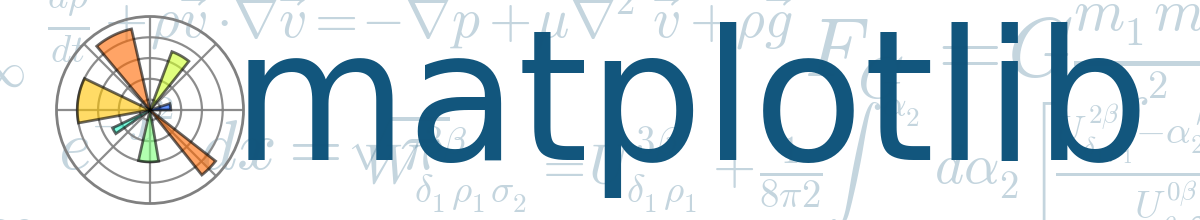# Data & Digital Scholarship Tutorials

## Workshop Description

Data Scripting: Python II: Leveraging Third-Party LibrariesThis workshop will cover importing libraries and other packages into Python scripts using Jupyter Notebooks on Google's Colaboratory platform.

Participants will work through a series of exercises in the following:

1. Installing libraries using pip
2. Importing installed libraries into Python scripts
3. Deep dives into (1) pandas, the Python Data Analysis Library, (2) matplotlib, a core data visualization library, and (3) spaCy, a natural language processing library

## Python II Workshop

(1) Take Workshops, (2) Pass Quizzes, (3) Become a Data Scholar

 Interested in becoming a Data Scholar?   Takes only six workshops! Pick any Two Categories Below, Take at Least Two Workshops from Each of Those Categories: (Total of 4)   Data Visualization Text Data Mining Python Data Scripting AND Pick any One Category Below, Take at Least Two Workshops from That Category:   (Total of 2) Research Data Management Finding Secondary Data

* Workshops are offered every semester. No need to fit all 6 in one semester. Become a Data Scholar at your own pace.

* Becoming a Data Scholar is not mandatory. Take any workshop you like.

 Head to Google Colaboratory Sign in https://colab.research.google.com Click New Python 3 Notebook   If you do not have this popup, click File/New Python 3 NotebookCommon pip commands:

• pip freeze
• pip search package
• pip install package
• pip uninstall package
 !pip freeze Displays all installed modules and their version!pip search twitterimport math help(math)To call a specific function... In a new code cell: Type math. After math, type a dot. If nothing happens, try hitting the tab key. select math.pi run cellhelp(math.degrees)QUIZ: How many degrees are 5 radians?

 Head to VADER Sentiment GitHub site https://github.com/cjhutto/vaderSentimentInstall VADER SentimentImport VADER Sentiment help(SentimentIntensityAnalyzer)sentences=['I hope everyone has a fantastic day!','I hate when Baylor loses football.'] from vaderSentiment.vaderSentiment import SentimentIntensityAnalyzer analyzer = SentimentIntensityAnalyzer() for sentence in sentences:     vs = analyzer.polarity_scores(sentence)     print(vs)Currency Converter is already installed in Google Colab   https://github.com/alexprengere/currencyconverterQuiz: Use Currency Converter to convert 70,000 USD to Euros.

 Matplotlib https://matplotlib.org/We will work with Pyplot. Click Tutorials Click Pyplot Tutorial from matplotlib import pyplot as plt It is standard to import pyplot as the shortened plt. All examples you find online will import pyplot as plt. Let's create a simple plot. from matplotlib import pyplot as plt x=[1,2,3,5] y=[5,11,20,25] plt.plot(x,y) To view the plot, add the following line and run the code block: plt.show()Add titleAdd labels for our axesAdd a second line with new y-values   from matplotlib import pyplot as plt x=[1,2,3,5] y=[5,11,20,25] z=[15,10,5,0] plt.plot(x,y) plt.plot(x,z) plt.title('My First Plot') plt.xlabel('x') plt.ylabel('y and z') plt.show()Add LegendBonus: plt.subplots() returns figure and an axes variables.   To adjust colors from default: fig, ax = plt.subplots() ax.set_prop_cycle(color=['red', 'green', 'blue'])   To save as an image: fig, ax = plt.subplots() fig.savefig('yourfilename.png')

 Pandas https://pandas.pydata.org/Import Pandas import pandas as pd Download practice csv table https://researchguides.baylor.edu/ld.php?content_id=51320019Upload to Colab    - Click the little tab on the left  - Click Files and upload practice_data.csvIn cell block, type pd.read and pause a moment until the list of structured file types are listed. (Make sure you run import pd first.) pd.read_csv('practice_data.csv')Store table in a dataframeIsolate a dataframe columnPlot column_a by column_b   plt.plot(practice_table.column_a,practice_table.column_b) plt.show()To add another plot, column a by column c:   Copy the plt.plot line and paste beneath it and change b to c.Quiz: Add a title to this plot

Compare population growth between the U.S. and China.

 Download world_pop.csv https://researchguides.baylor.edu/ld.php?content_id=51320021   Upload to Colabworld_data=pd.read_csv('world_pop.csv') world_data   To include all rows:   world_data=pd.read_csv('world_pop.csv') pd.set_option('display.max_rows', None) world_dataCreate a dataframe containing only data for the United States   us=world_data[world_data.country=='United States'] usQuiz: Create a dataframe containing only data for China called china Plot U.S. population by yearNotice the scientific notation? Adjust by dividing population by 1 million. plt.plot(us.year,us.population / 1000000) plt.show() Add China population by year to our plotComplete plot with title, labels, and legend.   plt.plot(us.year,us.population / 1000000) plt.plot(china.year,china.population / 1000000) plt.title('U.S. and China Population Growth') plt.xlabel('year') plt.ylabel('pop in millions') plt.legend(['U.S.','China']) plt.show()Adjust to show % population growth per year instead of raw population counts.   Plot % growth from the first year So year 1 will be 100%. Rest of the data will be % relative to year 1 New Code Cell us.population   First row is index number 0Values can be queried by index number location using iloc[]Divide each year of U.S. population by the first year's population, times 100 to calculate percent.In previous code cell, adjust plt.plot lines to show % change instead of raw counts.   plt.plot(us.year,us.population / us.population.iloc * 100) plt.plot(china.year,china.population / china.population.iloc * 100) plt.title('U.S. and China Population Growth') plt.xlabel('year') plt.ylabel('pop in millions') plt.legend(['U.S.','China']) plt.show()Natural Language Processing with spaCy https://spacy.io/Download trump2019sotu.txt https://researchguides.baylor.edu/ld.php?content_id=51320244Create a new Python 3 Notebook   and Upload to Colab Read text file into address variable f=open('trump2019sotu.txt','r') address=f.read() f.close() print(address) Create a new code cell   Import spacy and create an NLP object from address that we can run NLP functions agains. import spacy nlp=spacy.load('en') doc=nlp(address) print lemma form of each word   for token in doc:     print(token.text,token.lemma_)To see parts of speech instead of lemmas, change token.lemma_ to token.pos_   other options: lemma_ pos_ tag_ is_stopQuiz: Adjust the above code cell to show whether a word is a stop word or not

 Create a structured list of words, parts of speech, and stop word status   import spacy nlp=spacy.load('en') doc=nlp(address) word_list=[] for token in doc:     word_list.append([token.text,token.pos_,token.is_stop]) word_listNew code cell   Convert list to a pandas dataframe   import pandas as pd word_table=pd.DataFrame(word_list,columns=['text','pos','stopword']) word_tableWrite to csv tableCalculate token frequencies word_table['text'].value_counts()

Calculate the Flesch Reading Ease Score of Trump's 2019 State of the Union Address.

f=open('trump2019sotu.txt','r')